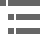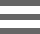## Depth of Field CalculatorExplore the approximate depth of field for common Nikon objectives with this interactive tutorial. Users have the ability to select from the current lineup of CFI60 infinity-corrected objectives as a function of aberration correction through the use of a pull-down menu.

To operate the tutorial, first select the appropriate objective from the Choose an Objective pull-down menu. The list covers all of Nikon's current objectives in the achromat, plan achromat, plan fluorite, and plan apochromat series. Several specialized objectives are included as well. Next, use the Choose e slider to vary the smallest distance (between a value of 4 and 24 microns) that can be resolved by a detector that is placed in the image plane of the microscope objective.

At high numerical apertures of the microscope, depth of field is determined primarily by wave optics, while at lower numerical apertures, the geometrical optical circle of confusion dominates the phenomenon. Using a variety of different criteria for determining when the image becomes unacceptably sharp, several authors have proposed different formulas to describe the depth of field in a microscope. The total depth of field is given by the sum of the wave and geometrical optical depths of fields as:

Where d(tot) represents the depth of field, l is the wavelength of illuminating light, n is the refractive index of the medium (usually air (1.000) or immersion oil (1.515)) between the coverslip and the objective front lens element, and NA equals the objective numerical aperture. The variable e is the smallest distance that can be resolved by a detector that is placed in the image plane of the microscope objective, whose lateral magnification is M. Using this equation, depth of field (d(tot)) and wavelength (l) must be expressed in similar units. For example, if d(tot) is to be calculated in micrometers, l must also be formulated in micrometers (700 nanometer red light is entered into the equation as 0.7 micrometers). Notice that the diffraction-limited depth of field (the first term in the equation) shrinks inversely with the square of the numerical aperture, while the lateral limit of resolution is reduced in a manner that is inversely proportional to the first power of the numerical aperture. Thus, the axial resolution and thickness of optical sections that can be attained are affected by the system numerical aperture much more so than is the lateral resolution of the microscope.

### Contributing Authors

Kenneth R. Spring - Scientific Consultant, Lusby, Maryland, 20657.

John C. Long and Michael W. Davidson - National High Magnetic Field Laboratory, 1800 East Paul Dirac Dr., The Florida State University, Tallahassee, Florida, 32310.

# Microscopy U - The source for microscopy education•••